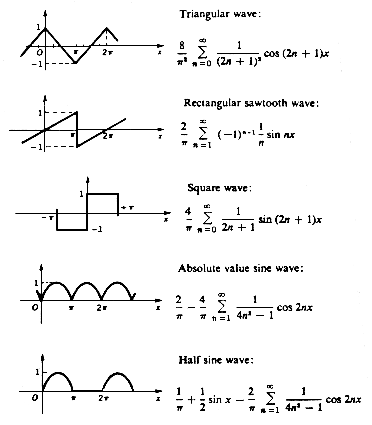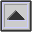FOURIER THEOREM

A mathematical theorem stating that a PERIODIC function f(x) which is reasonably continuous may be expressed as the sum of a series of sine or cosine terms (called the Fourier series), each of which has specific AMPLITUDE and PHASE coefficients known as Fourier coefficients.

The application of this theorem to sound is known as FOURIER ANALYSIS and FOURIER SYNTHESIS. The theorem was developed by the French mathematician J.B. Fourier around 1800.Fourier series of common WAVEFORMs.

home